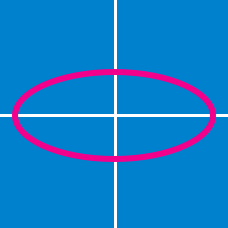Geometry

# Conics - Circle General Equation

What is the equation of the circle that passes through the origin, has its center on the line $x+y=4,$ and cuts the circle $x^2+y^2-6x+16y+24=0$ orthogonally?

Find the sum of all possible values of $b$ such that the two points $(4, 0 )$ and $(- 4, 0)$ lie on the circle $x^2 + y^2 + ax + by +c =0$ with radius $6$ .

If the line $y=ax+b$ cuts the circle $x^2+y^2-4x+8y-29=0$ into two equal parts, then what is the value of $2a+b ?$

If the circle $x^2+y^2+ax+by+c=0$ passes through three points $(-1,-8), (2,1), (6,-1),$ what is the value of $a+b+c ?$

If the circle $x^2+y^2+Ax+By+C=0$ passes through the origin and has the same center as the circle $x^2+y^2-9x+8y-3=0,$ what is the value of $A+B+C?$

×